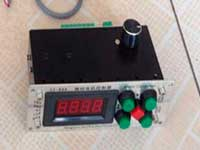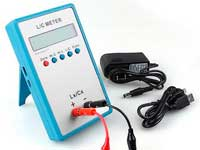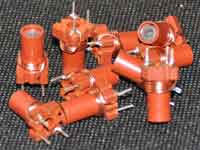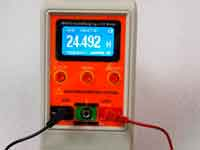Rating 0.00 (0 Votes)

Parallel resonant LC circuit

A parallel resonant circuit in electronics is used as the basis of frequency-selective networks. Real circuit elements have losses, and when we analyse the LC network we use a realistic model of the ideal lumped elements in which losses are taken into account by means of "virtual" serial resistances RL and RC.The Coil32 program calculates the losses in the wire coil (RL) without losses in former, shield, core and all objects interacting with the electromagnetic wave that is surrounding the coil. However, it takes into account the skin effect and proximity effect. Constructive quality factor of the coil - QL takes into account the same losses. This is not a Q-factor of the whole circuit, yet the Q-factor only of the coil, which is associated with its loss resistance by the equation:Losses in the capacitor an order of magnitude smaller and are characterized by the quality factor of the capacitor. Since capacitor losses are concentrated mainly in the dielectric, we can assume that its quality factor QC and the equivalent series loss resistance RC are associated with the parameters that take into account dielectric losses, as follows:At the analysis of this circuit, it is often converted to an equivalent parallel RLC-circuit. In this case, replacing resistances and reactants by conductances and admittances, we simplify the analysis and the resulting formulas are identical to formulas of the serial circuit. Many hams believe that the series RL and RC just converted to parallel R. It is not:As you can see resistance and reactance in this transformation are mixed, so for clarity, let us analyze without using of conductances directly on the source schema. The input impedance of the circuit looks as follows:Active and reactive (imaginary) components:At resonance the currents in the reactive elements (IL, IC) in Q times greater than the total circuit current (I), for a parallel circuit this phenomenon is called current resonance.

The resonant frequency of the parallel resonant circuit is the frequency at which the reactive component of input impedance is zero, the input impedance is only active, and, accordingly, the phase shift between current and voltage at the input terminals of a circuit is also equal to zero. Equating to zero Xi and after the appropriate transformations we get the following formula for the resonant frequency of the parallel oscillatory circuit:One of the most important parameters of the circuit is its characteristic resistance:

ρ = √L/C

The formula of resonance frequency can be represented in a different way:ω0 is the resonant frequency of the series resonant circuit with the same L and C.

As you can see the resonant frequency of the parallel LC-tank is equal to the resonant frequency of the series LC-circuit, composed of the same elements, with the addition of the correction factor: [(L/C - RL^2)/(L/C - RC^2)]. In practice, this ratio is always close to unity and equal to unity if RL=RC or RL=RC=0.

Example:

We have a circuit with inductance 3μH and capacitance 42pF, the resistance losses of the coil RL=2 Ohm, the capacitor losses is RC=0.1 Ω. According to Thompson formula the resonant frequency of the circuit is equal 14.178649 MHz, precisely calculating by the formula  — 14.178253 MHz. As you can see, resistance losses add more reactivity to a perfect LC-circuit, and move its resonance frequency down, in this case by almost 400 Hz.

This is a very small deviation should be borne in mind, but it is much smaller than the deviations introduced unaccounted parasitic capacitances. Therefore, on conditions that: RL<< ρ, RC<< ρ, which is usually the case in practice, we can assume that the conditions of current resonance in parallel circuit coincide with the conditions of voltage resonance in serial circuit, composed of the same elements L and C,

ω0=1/√LC or f0 = 1/(2π√LC)

Under the same conditions: RL<< ρ, RC<< ρwhere

• Zi-ser = RΣ + j(ωL - 1 ⁄ ωC) is the input impedance of the serial circuit, composed of the same elements.
• RΣ = RL + RC;

As you can see, we can assume that the loss resistance of the coil and capacitor are summed, so the total quality factor of the circuit Q can be determined by the following expression:At the resonant frequency ω0:Because reactances cancel each other, the circuit at the resonant frequency has only resistive impedance equal to Re (the effective resistance of the circuit)

It is important the parameter of bandwidth of a circuit. It's the frequency interval Δf within which the impedance Zi-par is not less than
1 ⁄ √2 (or 0,707) from maximum at the resonant frequency. Q-factor of the circuit and bandwidth are related by following expression:

Q = f0/Δf

Сomments from anonymous guests are enabled with moderation.

Coil Winding MachineUS \$187.53
The system consists of individual components including a controller, a stepping motor, a foot switch, power adapter and output shaft. This design makes it very easy for you to integrate the system into your own project.

LC Meter LC200AUS \$37.99
Measuring Capacitance Range:
0.01 pF - 100mF
Measuring Inductance Range:
0.001 uH - 100H
Measurement accuracy:1%.

RF InductorsUS \$6.43
Variable Ferrite Core Inductor coil set 3.5Turns 25-100MHZ

Auto Ranging LCR MeterUS \$63.07
Meter can measure 0.00pF to 100.00mF capacitance, 0.000uH to 100.00H inductance and 0.00? to 10.000M? resistance. Very suitable for small value capacitor and inductor measurement.